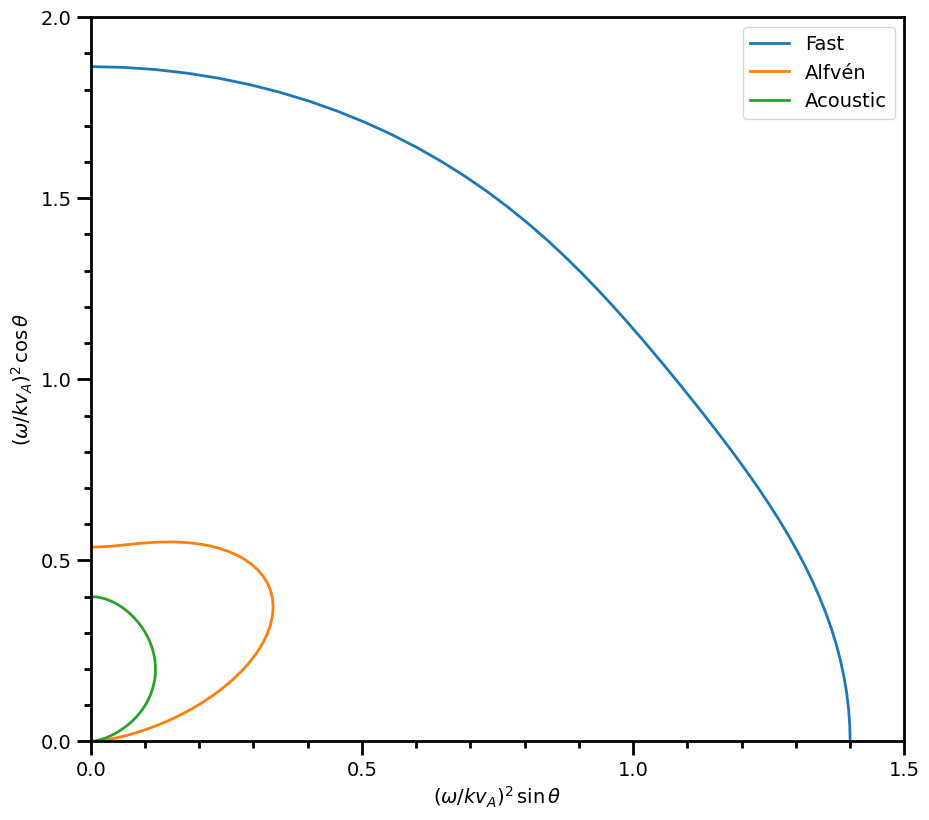# plasmapy.dispersion.two_fluid_dispersion¶

## Functions¶

 two_fluid_dispersion_solution(*, B, ion, k, …) Using the solution provided by Bellan 2012, calculate the analytical solution to the two fluid, low-frequency ($$\omega/kc \ll 1$$) dispersion relation presented by Stringer 1963. tfds_(*, B, ion, k, n_i, T_e, T_i, theta[, …])

## Example Notebooks¶